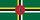1

## Dispatch → Account → Science

byThe Principality of Higara. . 4 reads.

## Energy Standards

Report on the current European Ecological Plan

The plan as of 2001

Reduction of carbon emissions by 15% by the end of 2005.
Hoped Comparative standard

2001 Energy production per type

Coal = 27%
Gas = 31%
Oil = 29%
Renewable energy = 13%

Cost of energy per kW/h = 0.52\$

Estimated in 2005 (Estime made in 2001)

Coal = 13%
Gas = 32%
Oil = 25%
Renewable energy =23%
Nuclear = 7%

Cost of energy per kW/h = 0.43\$

Actual Production in 2005 [From the Census & Statistics Bureux]

Coal = 21%
Gas = 19%
Oil = 32%
Renewable energy = 24%
Nuclear = 5%

Cost of energy per kW/h = 0.39\$

Estimated in 2010 [Estimes from 2005]

Coal = 14%
Gas = 21%
Oil = 23%
Renewable = 30%
Nuclear = 12%

Cost of Energy per kW/h = 0.28\$

Actual Production in 2010 [From the Census & Statistics Bureux]

Coal = 11%
Gas = 25%
Oil =26%
Renewable = 38%
Nuclear = [NUCLEAR PROGRAM SCRAPPED]

Cost of energy per kW/h = 0.26\$